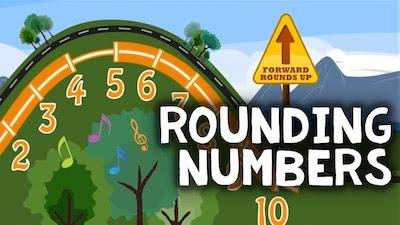# 3 Ways You Round Numbers Every Day

While rounding numbers may seem a strange phrase, the truth is that you may be surprised about its utility and use every single day.

One of the things that it is important to keep in mind is that most of the time, you will be rounding numbers that are decimals and not whole numbers. While it is possible to round whole numbers and it can be very useful, most of the time you will simply be rounding decimals.

## Rounding Numbers Rules

One of the methods that tend to be more frequently applied when you are looking to round numbers involves looking at the final digit and determine if it is equal or greater than 5 or if it is less than 5.

Us our free rounding calculator.

A decimal number is composed of an integer for the whole number to the left of the decimal point and the fractional part to the right of the decimal point.

So, when you want to round decimal numbers, you are actually trying to have fewer digits on the fractional part, which is the right of the decimal point. And this is simply to make it easier for you to make calculations. Besides, the numbers to the right of the decimal point usually show small amounts and sometimes it’s okay to ignore very small amounts.

But let’s take a look at some examples so that you can remember how to do this the right way.

Let’s imagine that you want to round the number 12.3742. Since the digit 4 is less than 5, then you will round 12.3742 to 12.37.

On the other hand, if you want to round the number 18.4592. Since the digit 9 is greater than 5, then you will round 18.4592 to 18.46.

Now that you already remembered how to round numbers, it0s time to see the numerous way you round numbers every day.

## #1: Taxes:

When you shop, do you pay a state sales tax? If the sales tax is 3% and you by something for \$56.30 how much sales tax do you pay?

\$56.30 X 0.03 = \$1.689

So, the tax is \$1.69.

Check out how to round numbers.

## #2: Do Mental Calculations:

Imagine that you and your friends go to a fast food restaurant. So, you want to ensure that you all have enough money with you. The best way to do it is to simply round the cost of each meal to the nearest dollar and add the amounts quickly and easily.

## #3: Get An Estimate:

Sometimes, you may need to round integers and not decimal numbers. As we already mentioned above, you can certainly do this using the same method described at the top.

Usually, you are interested in rounding to the nearest multiple of 10, 100, 1,000 or million. For example, in 2006 the census department determined that the population of New York City was 8,214,426. Since this number is hard to remember and if we say the population of New York City is 8 million it is a good estimate because it doesn’t make any real difference what the exact number is.